Previous: Working with files Up: Working with files Next: Using blockMesh generator

This is an automatically generated documentation by LaTeX2HTML utility. In case of any issue, please, contact us at info@cfdsupport.com.

## Notes on editing files in Linux

• For working with files in Linux, we have good experiences with following software; ordered with respect to how big action to take within the file.

1. mcedit (F3 or F4 in midnight commander)
2. gedit
3. nedit (for very big files)
4. kate
5. kdevelop

## Resolution

This parameter control the number of sampling faces in the slice. In the slice there will be approximately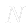faces in the axial direction. The faces are squares and their number in the radial direction is calculated automatically.

## turbulentMixingLengthDissipationRateInlet

zeroGradient boundary condition simply extrapolates the quantity to the patch from the nearest cell value. The meaning is, the quantity is developed in space and its gradient is equal to zero in direction perpendicular to the patch (perpendicular to the boundary). See figure (). U, k, omega, epsilon, T:
 boundaryField
{
".*_outlet"
{
}
}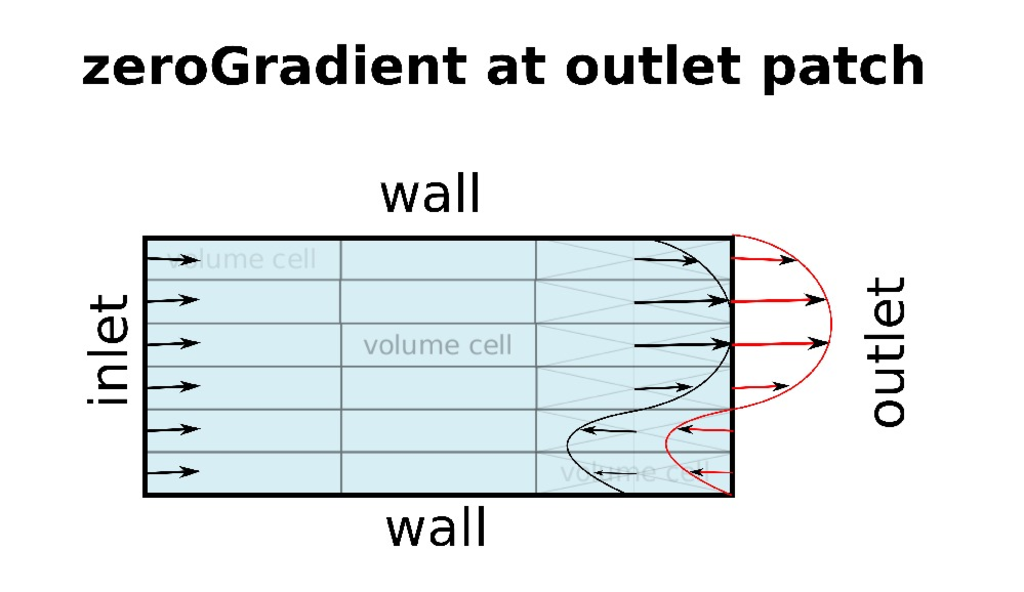Previous: Turbulence Up: TCFD – GUI Setup Next: Boundary condition: Outlet
This is an automatically generated documentation by LaTeX2HTML utility. In case of any issue, please, contact us at info@cfdsupport.com.

### Boundary condition: Inlet

Examples of inlet boundary conditions settings are displayed in Figuresand. It is possible to define an arbitrary number of inlet boundary conditions. They can be assigned to individual inlet patches defined in the section “Components”. There are several possible ways how to prescribe the desired behavior of the simulated fields at the inlet to the computational domain: Prescribing a fixed inflow (either in kg/s or m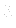/s), or requesting a fixed total pressure. The choice of the boundary conditions is done by selection of one item of the drop-down list “Inlet BC”. The rest of the panel is then adjusted to the user’s choice. Parameters of the turbulence model chosen in the section “Turbulence” are common to all boundary condition types. If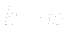or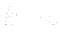model is used, then the parameters “Turbulent energy intensity” and “Turbulent dissipation” are available. If Spalart-Allmaras model is used, then “Turbulent eddy viscosity” is available.
• “Turbulent energy intensity” sets the fraction of the inlet turbulent and total kinetic energy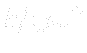, typically five per cent (0.05).
• “Turbulent dissipation” sets the inlet turbulent energy dissipation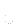within the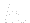models, or the inlet turbulent energy dissipation rate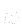within theturbulent model.
• “Turbulent eddy viscosity” sets the incoming value of the quantity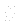.
The exclusive parameters of the “Mass flow rate” inlet boundary condition are:
• “Mass flow rate”.
The exclusive parameters of the “Volumetric flow rate” inlet boundary condition are:
• “Volumetric flow rate”.
The exclusive parameters of the “Total pressure” inlet boundary condition are:
• “Total pressure”, which is the inlet total pressure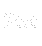and
• “Total temperature”, which is the inlet total temperature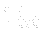(only needed and available for compressible simulations).
The exclusive parameters of the “Fixed velocity” inlet boundary condition are:
• “Fixed velocity”, which are the components of the velocity at the inlet.
The flow rate boundary conditions have also directed variants (“Directed mass flow rate” and “Directed volumetric flow rate”), which allow specification of the angle of the velocity vectors at inlet. This is done by setting the meridional and circumferential angle of the inlet direction. The inlet direction is a vector that points typically outside from the geometry and the velocity vectors will be oriented to be antiparallel with the inlet direction vectors (i.e. to point inwards). The meridional angle is the angle between the inlet direction vectors and the direction of the rotation axis. Allowed values of the meridional angle are between 0 and 180 degrees. The circumferential angle of the inlet direction vector at a given face is the angle between the inlet direction vector and the plane formed by the axis and the position vector of the given face. The range of the circumferential angles is from -90 degrees to +90 degrees, where positive angles correspond to positive orientation with respect to the rotation axis (right hand rule). Most of the dimensioned quantities have a user-selectable unit. Also, when the calculation is set up as transient and the advanced mode is switched on, then some of the boundary condition parameters accept a CSV file with time-dependent values of the parameter. The first column of the CSV file must contain the times, the second column must contain the values of the parameter (e.g. mass flow or total pressure), in the same units as chosen for the steady-state initial calculation. Clicking on “+ CSV” button will open a file selection dialog; alternatively, the path to the file can be put by hand into the edit field next to the button. When no time-dependent CSV data are specified, then the transient calculation will use the same value for the boundary condition parameter as the stationary calculation. If the calculation is set to run longer than what times are available in the CSV file, it will use the last available value of the parameter once the maximal time defined in CSV is exceeded.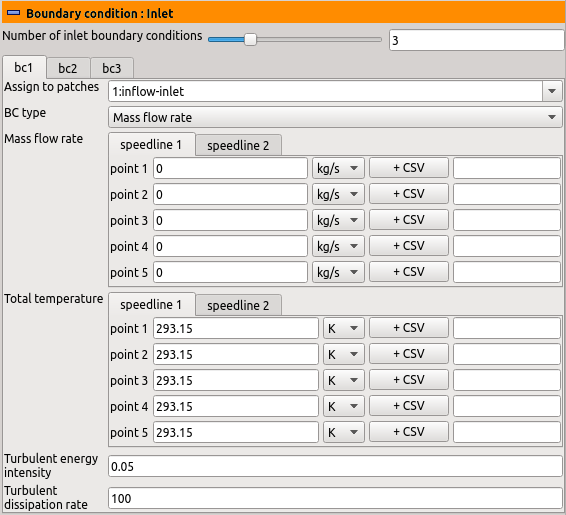Figure: Plugin TCFDSource – inlet boundary conditions: Mass flow rate.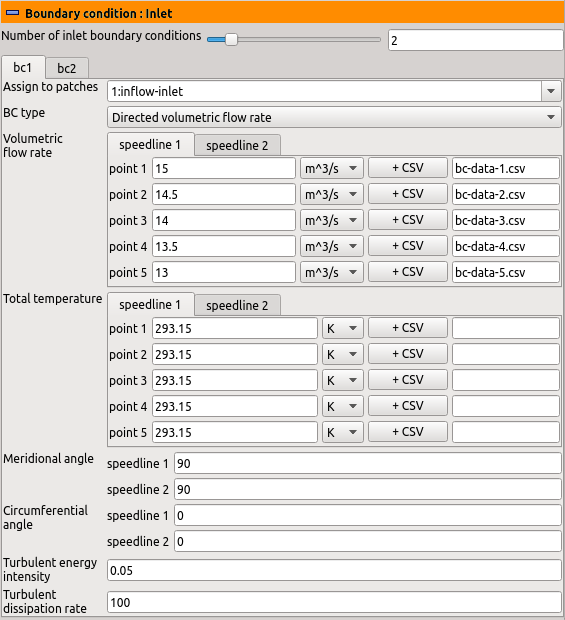Figure: Plugin TCFDSource – inlet boundary conditions: Directed volumetric flow rate.